• 国外bitcraze公司的UWB+IMU定位标签，融合了UWB和IMU，包括3轴加速度计/陀螺仪和DWM1000，低延迟2.4 GHz无线电，支持蓝牙LE，5-12V电源或micro-USB，可以通过USB、UART、低延迟2.4 GHz无线电或蓝牙LE进行数据通信和...
• ## 用IMU定位难点分析

千次阅读 2018-09-14 10:40:39
用IMU定位难点分析 IMU组成  一般IMU由加速度器acc、陀螺仪gyr组成，还可以外加一个磁力计mag... IMU定位原理  对于acc+gyr方式：acc+gyr得到比较准确姿态，去除acc重力分量影响；二次积分可以得到位置；  ...
用IMU定位难点分析

IMU组成
一般IMU由加速度器acc、陀螺仪gyr组成，还可以外加一个磁力计mag；

acc积分可以得到速度，再积分可以得到位置；静止时可以得到姿态方向；
gyr积分可以得到姿态方向；
mag本身就可以得到姿态方向；

IMU定位原理
对于acc+gyr方式：acc+gyr得到比较准确姿态，去除acc重力分量影响；二次积分可以得到位置；
对于acc+gyr+mag方式：需要acc+gyr+mag方式得到比较准确姿态，其他处理方式一样；

难点分析
IMU定位不准，主要由于2点造成：

a. 姿态不准导致重力影响去除不了，误差不断累积；
b. 器件本身漂移不能去除，如acc从静止到运动再到静止，acc积分得到的速度应该为0， 而实际不为0；
现对上述2点进行说明：

重力影响：可以采用一定的方法(如MahonyAHRS、MadgwickAHRS、Kalman，具体可参见xio)：对于acc+gyr方式，acc只能在静止时得到姿态，运动时不能，因而在运动情况下姿态不准确，重力影响去除不了；对于acc+gyr+mag，可以用mag得到姿态，提高姿态准确性，当有磁场干扰时，该方式效果也存在问题；
本身漂移：对于此问题，一般的处理方法为用高通滤波去除，但高通滤波后得到的只是相对运动关系，不能得到准确的位置信息；因而再实际应用中可以根据实际情况设计相应的处理方法，如步态定位中，可以采用运动静止判断，如下图所示为步态数据运动静止判断矫正后的效果：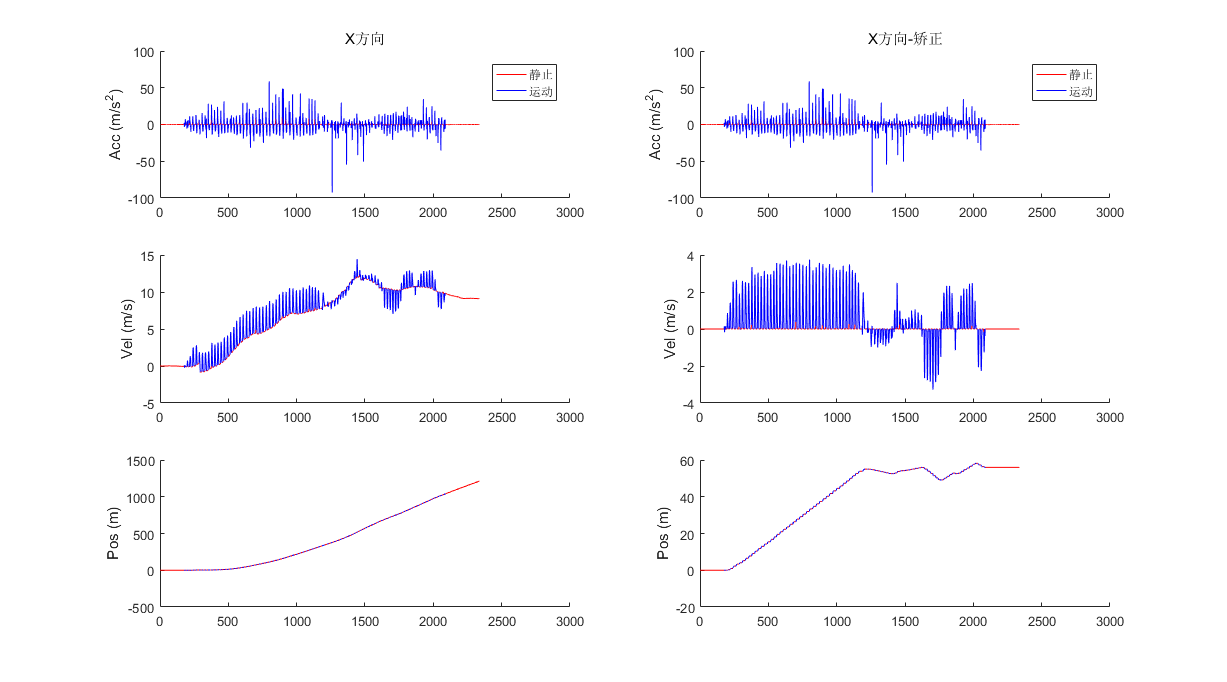应用
IMU定位存在不可避免的漂移，需针对具体应用设计相应的处理算法。
目前使用较多的步态定位。


展开全文• 这是一个关于纯imu定位的matlab代码，考虑地球自转，自带误差分析，有助于了解惯导定位原理，特别是累积误差
• 加速度计基本原理 核心：牛顿第二定律 一、mems加速度计基本原理 加速度计基本结构如上图，由上电容、中电容板(可移动)、下电容板等组成；当加速度达到一定值后，中电容板会移动，与上、下电容板的距离就会...
加速度计基本原理

核心：牛顿第二定律

一、mems加速度计基本原理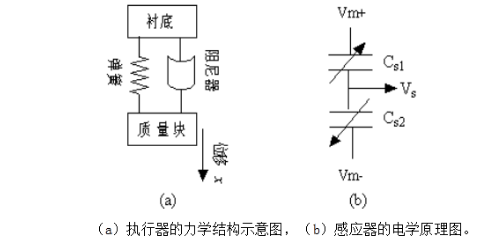加速度计基本结构如上图，由上电容、中电容板(可移动)、下电容板等组成；当加速度达到一定值后，中电容板会移动，与上、下电容板的距离就会变化，上、下电容因此变化。电容变化跟加速度成比(如下面公式)，通过对输出电压数字处理后，输出数字化信号。

电路公式推导如下：

（1）由平行极板电容基本公式：

得到上下极板与移动板构成的电容大小与位移量的关系如下：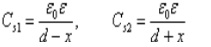（2）从右图的电路示意图可得：

对于两个电容串联，电容分压公式：

设总电压是U，C1、C2上的电压分别是U1、U2，则

U1=C2*U/(C1+C2)

U2=C1*U/(C1+C2)

对于两个电容串联，公式演变为：

其中，vm为输入电压

（3）由（1）（2）得到的公式，可以写出：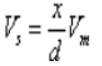（4）根据牛顿第二定律、胡克定律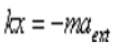（5）整理得，加速度与输出电压有如下关系：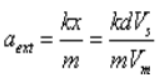以上摘自：惯性导航之MEMS加速度计原理

二、加速度计与坐标系

1、想象加速度计内部为一个圆球在一个方盒子中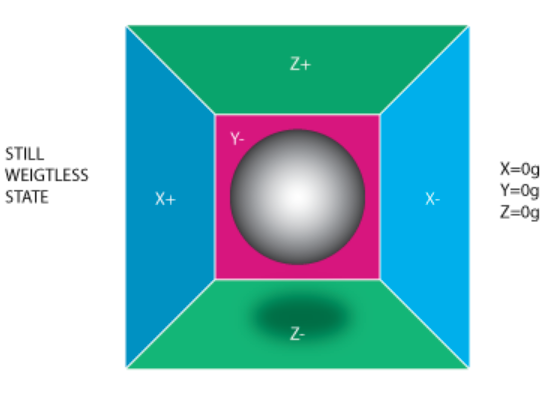如果盒子不受重力场或其他力场的影响，那么球将处于盒子正中央。

下面给XYZ+-共6个方向分配一个测量压力的单元，如果盒子以加速度g突然向左移动（如下图），那么球将撞上X-墙，X-墙测得压力，X轴输出值-1g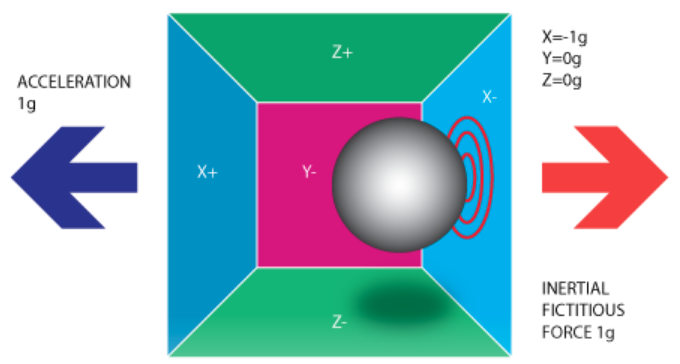2、现在假设模型受到重力场的影响，那么球会落在Z-面上，Z轴测得-1g，在这种情况下盒子没有移动但我们任然读取到Z轴有-1g的值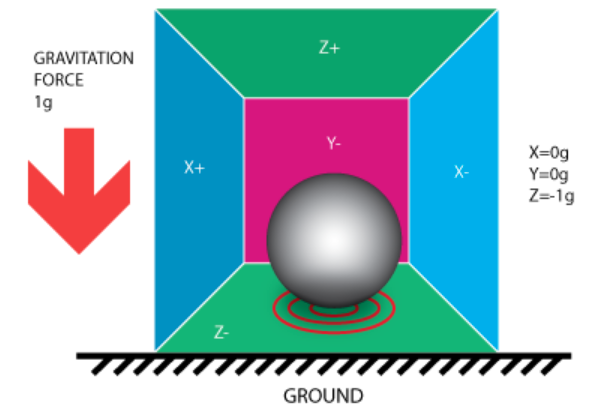3、以上模型是加速度计的基本模型，关于合加速度与三个轴的夹角关系如下分析：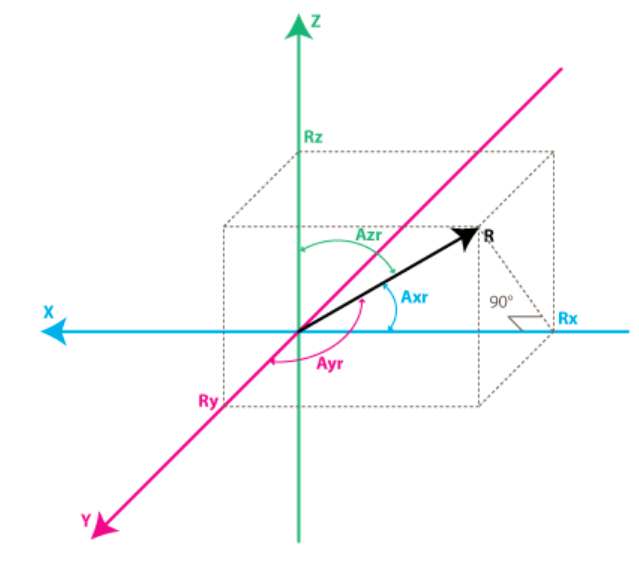设合加速度方向的矢量为R，各个轴上的分量分别为Rx，Ry，Rz，则有：

所以，有：

摘自：A Guide To using IMU (Accelerometer and Gyroscope Devices) in Embedded Applications.


展开全文• ## IMU&GPS融合定位：：IMU姿态解算

千次阅读 多人点赞 2019-11-16 21:36:47
另外，姿态解算不仅仅用于无人机领域，无人车领域也需要进行姿态解算，用以进行GNSS和IMU、激光点云的融合定位。 2. 主要内容 传感器基本原理 坐标系描述 姿态的几种表示方式 姿态解算的基本算法 3. 传感器基本...
姿态解算
1. 背景
姿态解算是飞控的一个基础、重要部分，估计出来的姿态会发布给姿态控制器，控制飞行平稳，是飞行稳定的最重要保障。另外，姿态解算不仅仅用于无人机领域，无人车领域也需要进行姿态解算，用以进行GNSS和IMU、激光点云的融合定位。

2. 主要内容

传感器基本原理
坐标系描述
姿态的几种表示方式
姿态解算的基本算法

3. 传感器基本原理

不展开，推荐以下参考：

AHRS姿态解算说明(加速度+陀螺仪+磁力计原理及原始数据分析)
IMU&GPS融合定位：：加速度计基本原理
……

4. 坐标系描述
运载体在三位空间中运动包含六个自由度，既有角运动又有线运动。在地球表面附近，运载体的角运动又有线运动。运载体上的惯性传感器（imu）是相对于惯性空间进行测量的，另外，地球绕其自转轴相对于惯性空间以常值角速度旋转，运载体在地球表面上的位置变化会引起其相对于地心的角速度变化。
4.1 地心惯性坐标系(i系,inertial frame)
地心惯性坐标系用oixiyizi表示，原点为地球中心，x轴指向春分点（赤道面与黄道面的交线再与天球相交的交点之一），春分点是天文测量中确定恒星时的起点，z轴为地球自转轴，指向北极，惯性传感器的输出就是基于该坐标系为参考坐标系的。
4.2 地球坐标系(e系,earth frame)
地球坐标系用oexeyeze表示，x轴指向本初子午线与赤道面的交点，z轴为地球自转轴，指向北极。e系与地球固连，也称 地心地固坐标系 （Earth-Centered Earth-Fixed,ECEF),地球坐标系相对于惯性坐标系的角速度大小为地球自传角速度，
$w_{ie}=7.2921151467*10^{-5} rad/s$
4.3 地理坐标系(g系,geographic frame)
WGS-84
4.4 当地导航坐标系(e系,earth frame)
导航坐标系用oexeyeze，它是惯性导航系统在求解导航参数时采用的参考坐标系。纯惯导系统的高度通道在原理上是发散的，因而惯导系统多采用当地水平坐标系作为参考坐标系，以实现水平和高度通道的解偶，即利用惯导长时间导航定位时只进行水平定位结算，简单地将高度值设为固定。
常用的导航坐标系为东-北-天（ENU）导航坐标系

X轴：指东
Y轴：指北
Z轴：指天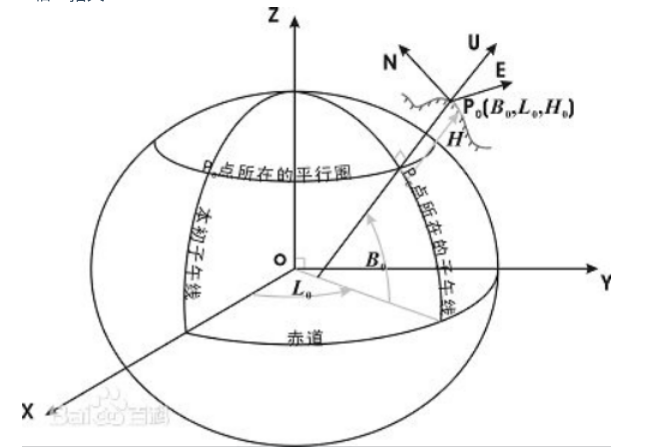4.5 载体坐标系(b系,body frame)
载体坐标系用obxbybzb表示，原点为载体重心，右-前-上坐标系描述：x轴沿载体横轴向右，y轴沿载体纵轴向前（即载体前进方向），z轴沿载体立轴向上。b系与载体固连，载体坐标系b系与导航坐标系n系的关系可用一组欧拉角表示。
4.6 常用相连坐标系(特指载体坐标系和导航坐标系))
4.6.1 东北天(ENU)——右前上
(1)东北天——当地导航坐标系

X轴：指东;
Y轴：指北;
Z轴：指天。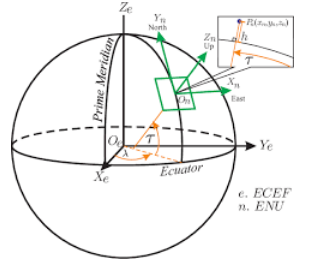画成平面，如下图：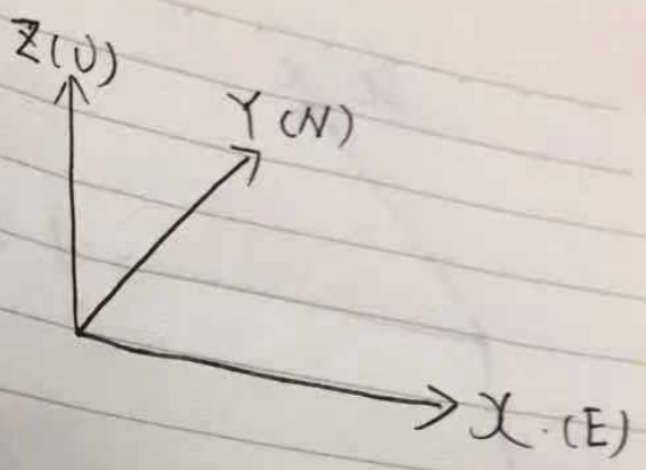(2)右前上——当地导航坐标系

X轴：指向载体右侧;
Y轴：指向载体前进方向;
Z轴：指天。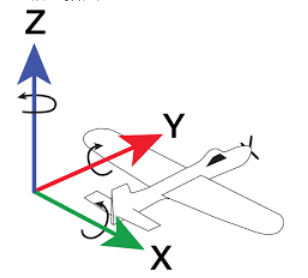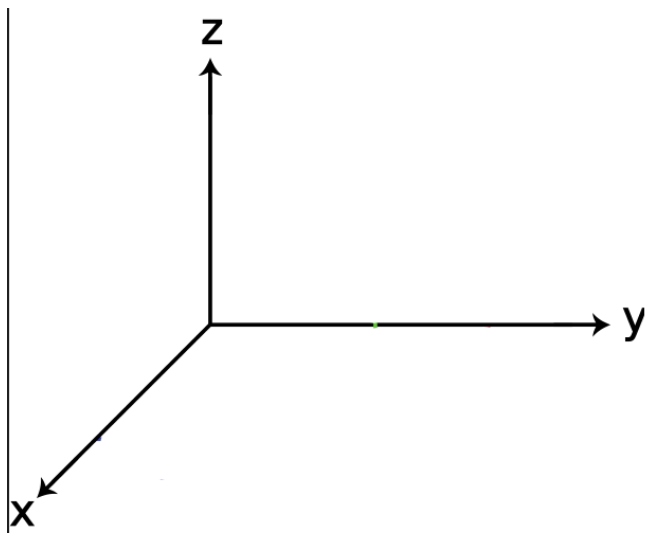5. 姿态的描述
5.1 欧拉角

欧拉角用来直观表示载体的姿态，如下图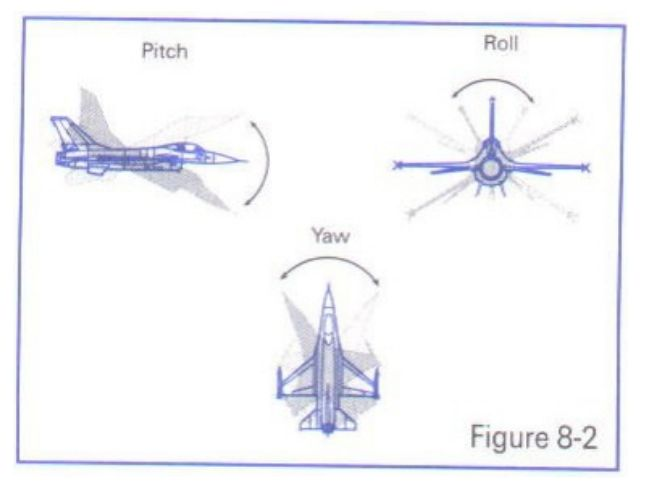偏航角Yaw $(\psi)$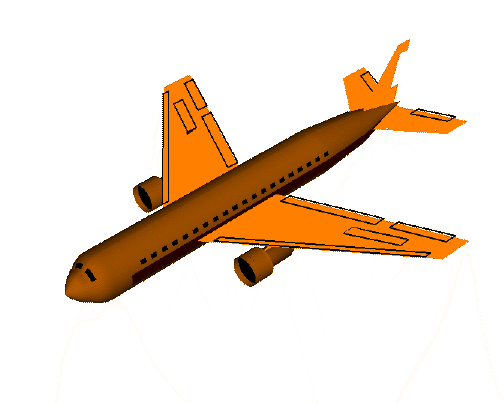俯仰角Pitch $(\theta)$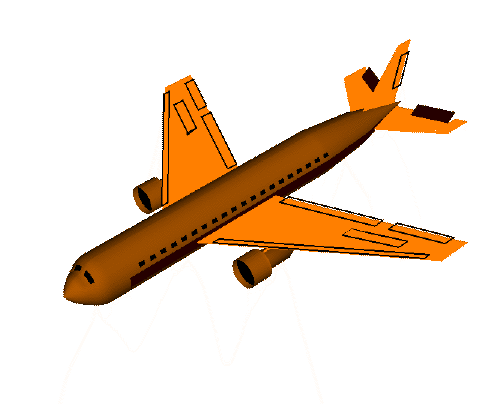翻滚角Roll $(\phi)$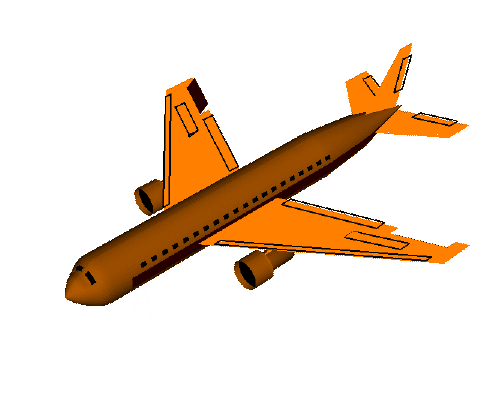Proper Euler angles (z-x-z, x-y-x, y-z-y, z-y-z, x-z-x, y-x-y)
指第一次旋转和第三次旋转中使用相同的轴（313），比较少用
泰特-布莱恩角
泰特布莱恩角表示围绕三个不同轴的旋转，如Z-X’-Y’’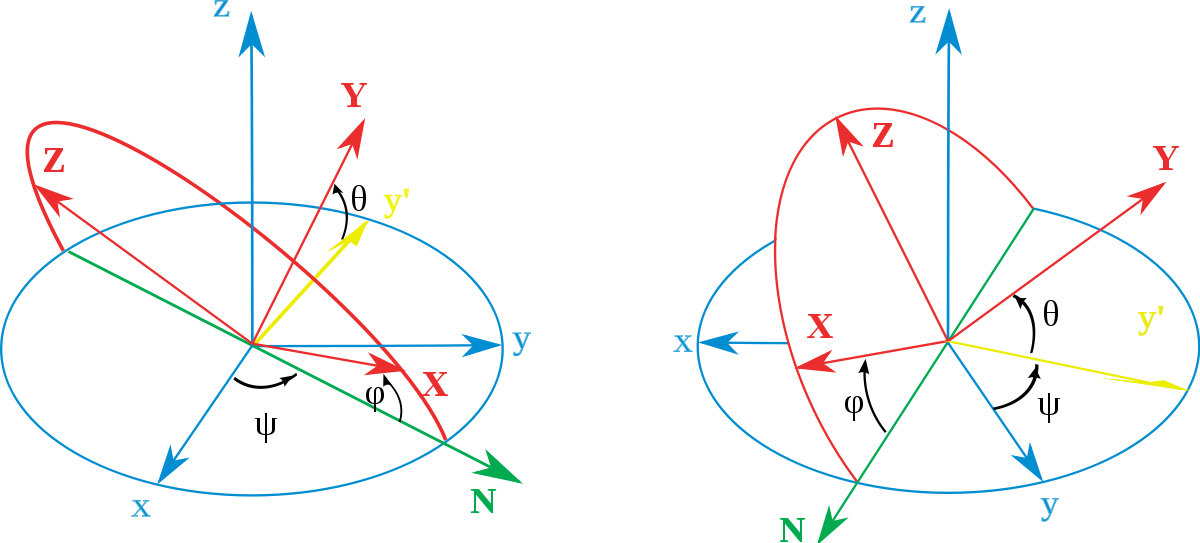上图描述了一组欧拉角，主要看右图就好了
(1)原坐标系1如蓝色的x-y-z
(2)坐标系1沿z轴，逆时针旋转$\psi (\psi >0)$，认为沿z轴逆时针旋转为正方向，$\psi=Yaw$；然后得到了黄色的坐标系2x’-y’-z’
(3)将坐标系2沿x(N)轴逆时针旋转$\theta(\theta >0)$，得到了红色的坐标系N-Y-Z
(4)将红色坐标系沿Y轴顺时针旋转$(\phi)$，得到了目的坐标系:红色的X-Y-Z坐标系

5.2 旋转矩阵（方向余弦矩阵)

由于使用欧拉角描述姿态存在万向节死锁的问题，具体参见：万向节死锁 gimbal lock
因此引入方向余弦矩阵

5.2.1 方向余弦矩阵的基本形式
一个向量的方向（姿态）我们可以用他在参考坐标系（地理坐标系）各个轴向的夹角的余弦来表示（及在各个轴的投影）。
类似的 一个坐标系 可以看成是3个向量组成，所以三个向量分别在坐标轴上的投影可以用来表示一个坐标系与参考坐标系的关系。这总共9个方向余弦组成了一个三阶矩阵，其对应方式如下图。
$\quad C_b^n= \begin{bmatrix} c_{11} & c_{12} &c_{13} \\ c_{21} & c_{22} &c_{23} \\ c_{11} & c_{12} &c_{13} \end{bmatrix} \quad$
其中，第 i 行、 j 列的元素表示参考坐标系 i 轴和姿态坐标系 j 轴夹角的余弦。事实上方向余弦和欧拉角没有本质区别，因为方向余弦实际上就是用欧拉角表示的。
5.2.2 方向余弦矩阵的举例推导
一个二维的坐标变换如下：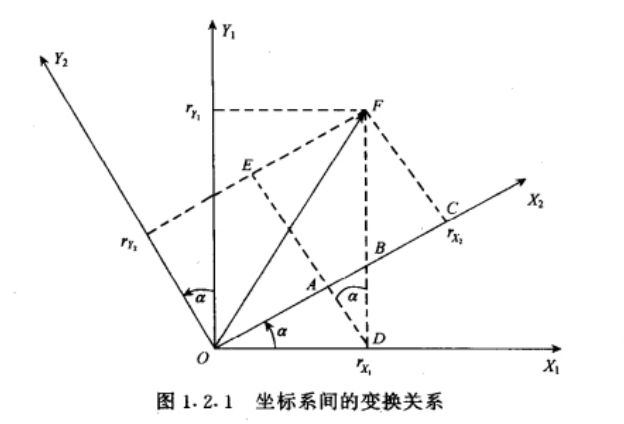点F为固定点，在坐标系1下的表示为$F(r_{x1},r_{y1})$，在坐标系2下为$F_{2}(r_{x2},r_{y2})$
由图可知
\begin{aligned} r_{x2}&=r_{x1}*\cos \alpha+ r_{y1}*\sin \alpha \\ r_{y2}&=r_{x1}*(-\sin \alpha)+r_{y1}*\cos \alpha \end{aligned}
推广到三维的情况下，可看作是原坐标系绕Z轴逆时针旋转，并写成矩阵形式：

（值得注意的是）:这个形式是原坐标系绕Z轴逆时针旋转，请不要看成了是 ‘点’绕Z轴逆时针旋转。

$\quad \begin{bmatrix} r_{x2} \\ r_{y2} \\ r_{z2} \end{bmatrix}= \begin{bmatrix} \cos \alpha & \sin \alpha &0 \\ -\sin \alpha & \cos \alpha &0 \\ 0 & 0 &1 \end{bmatrix} \begin{bmatrix} r_{x1} \\ r_{y1} \\ r_{z1} \end{bmatrix} \quad$
由上面的例子，可推理余弦矩阵各个元素的意义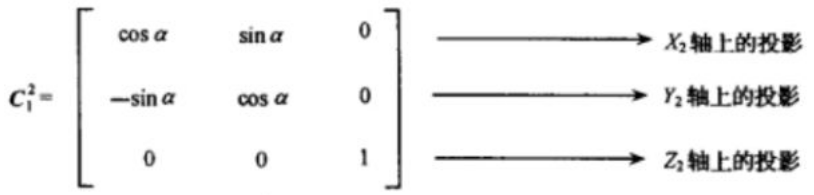第1行表示旋转之后的X2轴在原坐标系(X1,Y1,Z1)轴下的投影
第2行表示旋转之后的Y2轴在原坐标系(X1,Y1,Z1)轴下的投影
第3行表示旋转之后的Z2轴在原坐标系(X1,Y1,Z1)轴下的投影

5.2.2 东北天ENU---->右前上的余弦矩阵$C_{n}^{b}$推导
假设我们现在有一个东北天坐标系和一个载体坐标系，现需要将东北天坐标系经过3次旋转，使得最终得到的坐标系与载体坐标系重合。

在此之前，需要做出一些规定

旋转的正方向为：从旋转轴看的逆时针方向
旋转的顺序为：Z-X-Y
对应的欧拉角：Yaw-Pitch-Roll

1. 绕Z轴逆时针旋转$\psi$——Yaw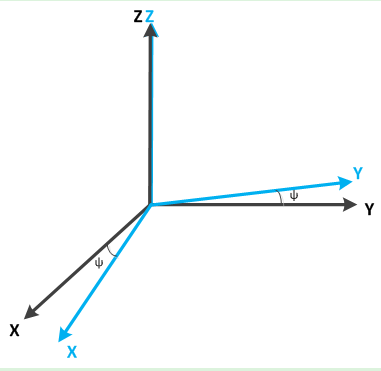得到旋转矩阵$C_{n}^{1}$
$\quad C_{n}^{1}= \begin{bmatrix} \cos \psi & \sin \psi &0 \\ -\sin \psi & \cos \psi &0 \\ 0 & 0 &1 \end{bmatrix} \quad$
2. 绕X’轴逆时针旋转$\theta$——Pitch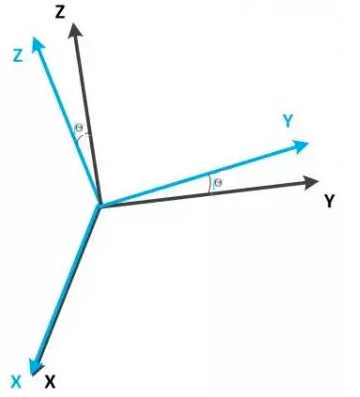得到旋转矩阵$C_{1}^{2}$
$\quad C_{1}^{2}= \begin{bmatrix} 1 & 0 &0 \\ 0 &\cos \theta & \sin \theta \\ 0 &-\sin \theta & \cos \theta \end{bmatrix} \quad$
3. 绕Y’'轴逆时针旋转$\phi$——Roll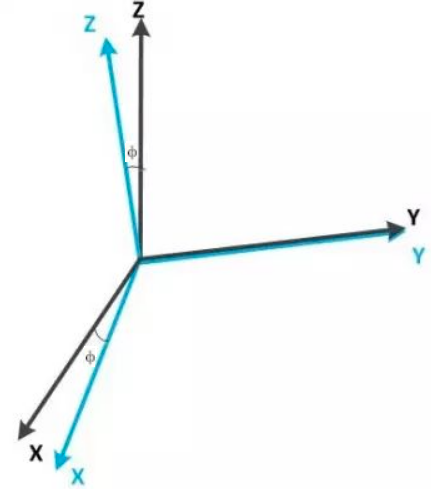得到旋转矩阵$C_{2}^{3}$
$\quad C_{2}^{3}= \begin{bmatrix} \cos \phi & 0 &-\sin \phi \\ 0 & 1& 0 \\ \sin \phi & 0& \cos \phi \end{bmatrix} \quad$
4. 得到ENU导航坐标系到右前上载体坐标系的方向余弦矩阵$C_{n}^{b}$
\begin{aligned} C_{n}^{b}=&C_{2}^{3}C_{1}^{2}C_{n}^{1} \\ =& \begin{bmatrix} \cos \phi & 0 &-\sin \phi \\ 0 & 1& 0 \\ \sin \phi & 0& \cos \phi \end{bmatrix} \begin{bmatrix} 1 & 0 &0 \\ 0 &\cos \theta & \sin \theta \\ 0 &-\sin \theta & \cos \theta \end{bmatrix} \begin{bmatrix} \cos \psi & \sin \psi &0 \\ -\sin \psi & \cos \psi &0 \\ 0 & 0 &1 \end{bmatrix} \\ =& \begin{bmatrix} \cos \psi \cos \phi -\sin \psi \sin \theta \sin \phi & \sin \psi \cos \phi+\cos \psi \sin \theta \sin \phi & -\cos \theta \sin \phi \\ -\sin \psi \cos \theta & \cos \psi \cos \theta & \sin \theta \\ \cos \psi \sin \phi+ \sin \psi \sin \theta \cos \phi & \sin \psi \sin \phi- \cos \psi \sin \theta \cos \phi & \cos \theta \cos \phi \end{bmatrix} \\ = & \begin{bmatrix} c_{11} & c_{12} &c_{13} \\ c_{21} & c_{22} &c_{23} \\ c_{11} & c_{12} &c_{13} \end{bmatrix} \end{aligned}

这里补一个按Z-Y-X顺序的旋转矩阵$C_n^b$5.3 四元数

用欧拉角表示姿态需要指定顺序和方向，用余弦矩阵表示姿态则参数较多，四元数出现了。
四元数（Quaternions）是由爱尔兰数学家哈密顿（William Rowan Hamilton）在1843年提出。

三维空间的任意旋转，都可以用绕三维空间的某个轴旋转过某个角度来表示，即所谓的Axis-Angle表示方法。这种表示方法里，Axis可用一个三维向量(x,y,z)来表示，θ可以用一个角度值来表示，直观来讲，一个四维向量(θ,x,y,z)就可以表示出三维空间任意的旋转。注意，这里的三维向量(x,y,z)只是用来表示axis的方向朝向，因此更紧凑的表示方式是用一个单位向量来表示方向axis，而用该三维向量的长度来表示角度值θ。

简单来说，四元数的思想就是把方向余弦矩阵的三次旋转表示为只绕一个旋转轴旋转一次完成，因此可以用4个数来表示这个过程，其中包括旋转轴向量的长度(θ)和旋转轴单位向量(x,y,z)

5.3.1 四元数的表示
\begin{aligned} \begin{bmatrix} q_0 \\ q_1 \\ q_2 \\ q_3 \end{bmatrix} = \begin{bmatrix} \cos \frac{\theta}{2} \\ x*\sin \frac{\theta}{2} \\ y*\sin \frac{\theta}{2} \\ z*\sin \frac{\theta}{2} \\ \end{bmatrix} \end{aligned}
5.3.2 四元数的复数定义

q=q0+q1i+q2j+q3k=[s,v]
其中 q0,q1,q2,q3均为实数, s=q0,v=[q1,q2,q3]，i2=j2=k2=−1
对于 i,j,k 本身的几何意义可以理解为一种旋转，其中 i 代表 x 轴与 y 轴相交平面中 x 轴正向向 y 轴正向的旋转， j 旋转代表 z 轴与 x 轴相交平面中 z 轴正向向 x 轴正向的旋转，k 旋转代表 y 轴与 z 轴相交平面中 y 轴正向向 z 轴正向的旋转， −i,−j,−k 分别代表 i,j,k 的反向旋转。

5.3.3 四元数的模
\begin{aligned} |q|=\sqrt{q_{0}^{2}+q_{1}^{2}+q_{2}^{2}+q_{3}^{2}} \end{aligned}
5.3.4 四元数的运算

主要运算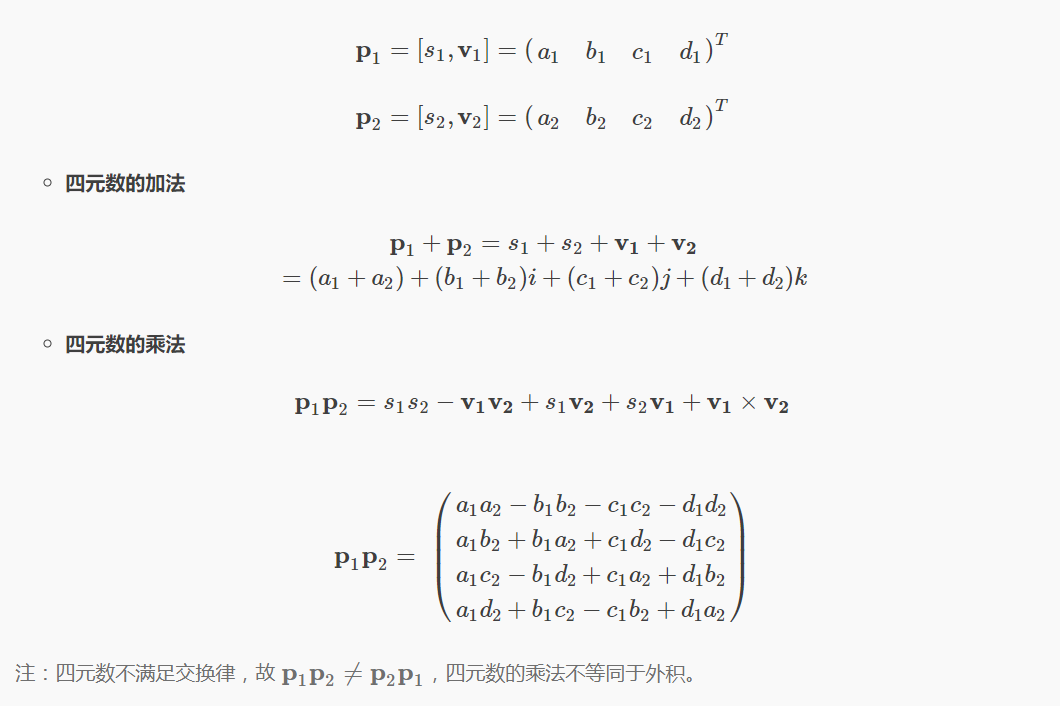四元数乘法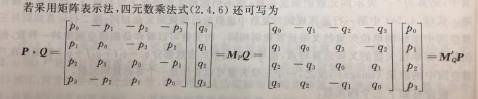乘法性质

满足结合律
不满足交换律
乘积的模等于模的乘积
乘积的逆等于各个四元数的逆以相反的顺序相乘

其他运算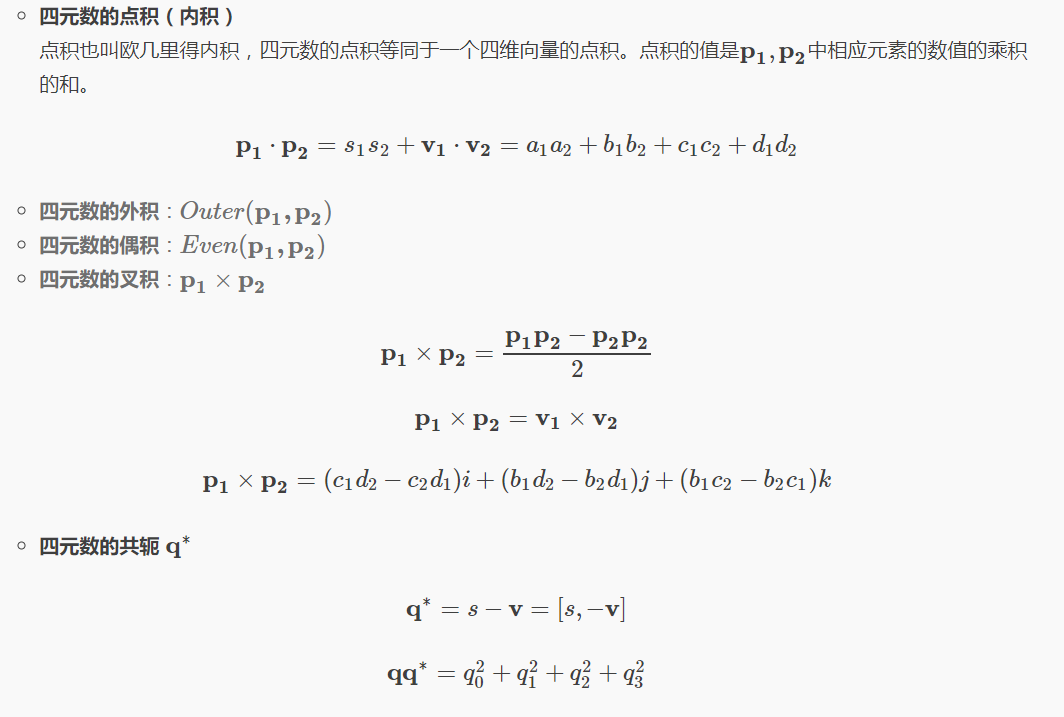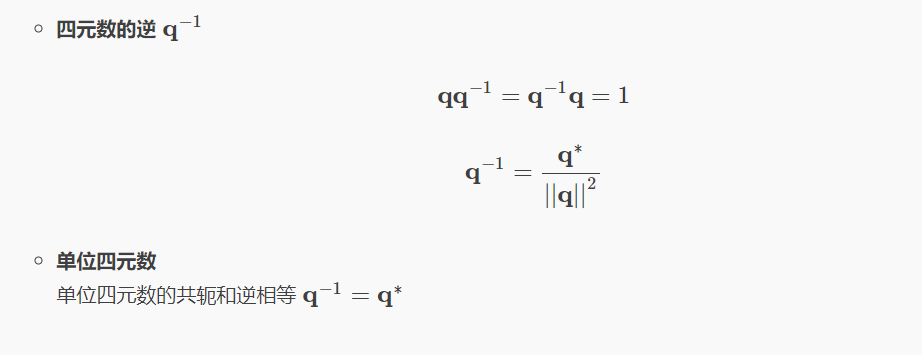*四元数部分参考：旋转矩阵、欧拉角、四元数理论及其转换关系

6. 姿态解算
6.1 传感器坐标系准备

在进行解算之前，需要完成十分重要的一步(但是很多资料都没展开描述)，将加速度计、陀螺仪、磁力计对其到右前上坐标系。原因是一些集成的imu中三者坐标系并不一致。

6.1.1 加速度计

车头朝前
向前加速，y轴加速度输出为+
向右加速，x轴加速度输出为+
向上加速，z轴加速度输出为+

6.1.2 陀螺仪

车头朝前
从Z+看向Z-(即从上往下看)，逆时针旋转，Z轴角速度输出为+
从X+看向X-(即从车右侧看)，逆时针旋转(车头上翘)，X轴角速度输出为+
从Y+看向Y-(即从车头看)，逆时针旋转，Y轴角速度输出为+

6.1.3 磁力计

车头朝北，即Y轴指北
磁力计应该输出形如
\begin{aligned} Mag_b= \begin{bmatrix} 0 \\ M_N \\ M_D \end{bmatrix} \end{aligned}
其中，
\begin{aligned} M_D <0 ，北半球 \\ M_D >0 ，北半球 \end{aligned}
将车头稍微向西偏
检查此时输出，正确的输出形如(主要看之前为0的那一项的变化)：
\begin{aligned} Mag_b= \begin{bmatrix} >0 \\ a*M_N \\ b*M_D \end{bmatrix} \end{aligned}
如果反向了，则需要添加‘-’号

6.2 初始姿态计算

在进行姿态解算前，需要确定初始的姿态，

使用加速度计确定俯仰和翻滚，pitch 和  roll
使用磁力计确定偏航角  yaw

6.2.1 确定俯仰和翻滚

对载体坐标系下的加速度(加速度计的输出)进行归一化
\begin{aligned} Acc=Norm(Acc) \end{aligned}
计算俯仰pitch
由于我们是以右前上作为载体坐标系，Y轴为车头方向，与网上很多飞控采用的前右下坐标系不一样，因此pitch与y轴方向加速度相关
\begin{aligned} pitch=atan2(acc_y,\sqrt{acc_x^{2}+acc_z^{2}}) \end{aligned}
计算翻滚roll
\begin{aligned} roll=-atan2(acc_x,acc_z) \end{aligned}

实现代码C++
Vector3d getPoseFrom_Acc(Vector3d _acc){
Vector3d acc=_acc;
acc.normalize();
double norm=sqrt(acc*acc+acc*acc+acc*acc);
Vector3d result;
result<<0,atan2(acc,sqrt(acc*acc+acc*acc)),-atan2(acc,acc);
return result;
}

6.2.2 确定偏航角

确定偏航角需要使用磁力计，使用磁力计之前请先按照官方给定校正说明对磁力计进行校正(另外还需要时不时校正，这个东西太弱了))

我们使用ENU和右前上坐标系，因此，当载体坐标系b系与导航坐标系n系重合时，即车头朝北时，正常输出如下：
\begin{aligned} Mag_b=Mag_n= \begin{bmatrix} 0 \\ M_N \\ M_D \end{bmatrix} \end{aligned}
两坐标系不重合时，磁力计输出如下：
\begin{aligned} Mag_{b1}= \begin{bmatrix} mag_x^{b} \\ mag_y^{b} \\ mag_z^{b} \end{bmatrix} \end{aligned}
假定有从导航坐标系n系到载体坐标系b系的方向余弦矩阵Cnb，那么将此时磁力计输出变换到导航坐标系下，得到:
\begin{aligned} Mag_{b1}=C_n^{b}Mag_n=C_n^{b} \begin{bmatrix} 0 \\ M_N \\ M_D \end{bmatrix}= \begin{bmatrix} mag_x^{b} \\ mag_y^{b} \\ mag_z^{b} \end{bmatrix} =& \begin{bmatrix} M_N (\sin \psi \cos \phi + \cos \psi \sin \theta \sin \phi)-M_D \cos \theta \sin \phi \\ M_N \cos \psi \cos \theta +M_D \sin \theta \\ M_N (\sin \psi \sin \phi- \cos \psi \cos \phi \sin \theta)+M_D \cos \theta \cos \phi \end{bmatrix} \end{aligned}
对上式进行变换:
\begin{aligned} my=M_N\sin \psi=&mag_x^{b}\cos \phi+ mag_z^{b}\sin \phi \\ mx=M_N\cos \psi=&mag_x^{b}\sin \phi \sin \theta+ mag_y^{b}\cos \theta -mag_z^{b}\cos \phi \sin \theta \end{aligned}
综上，可得偏航角Yaw
\begin{aligned} Yaw=\psi=atan2(my,mx) \end{aligned}
6.3 基于Mahony算法的姿态解算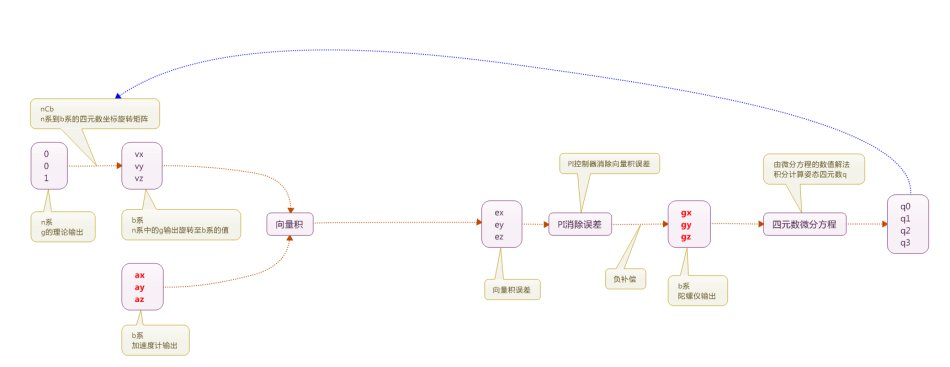数据准备

取初始姿态Yaw,pitch,roll
利用euler_312_To_quaternion()函数，得到初始姿态对应的四元数q
利用C_nb_312_from_qnb()函数，得到初始姿态对应的旋转矩阵Cnb

利用磁力计校正Yaw偏差计算

取当前b系下磁力计输出,并归一化
$Mag=Norm(Mag)$
将b系的磁力向量转换到n系下
$MagRef=(C_{n}^{b})^TMag$
构建基于b系磁力分量和姿态矩阵的理想磁力计输出
\begin{aligned} MagRef\_= & \begin{bmatrix} 0 \\ \sqrt{MagRef^2+MagRef^2} \\ MagRef \end{bmatrix} \\ MagDir\_hat=& \frac{1}{2}C_{n}^{b}MagRef\_ \end{aligned}
利用叉乘计算实际与理想的偏差
$Mag\_err=Mag \times MagDir\_hat$
设置磁力分量偏差的Kp和Ki值
计算磁力分量误差
\begin{aligned} err_p=&kp_{yaw}*Mag\_err \\ err_i=&ki_{yaw}*\Delta T*Mag\_err \end{aligned}

利用加速度计校正pitch、roll偏差计算

取当前b系下加速度计输出,并归一化
$Acc=Norm(Acc)$
由于标准n系下的加速度计输出应该只有重力分量，即归一化后的理想n系加速度为:
\begin{aligned} Acc_n= \begin{bmatrix} 0 \\ 0 \\ 1 \end{bmatrix} \\ Acc\_hat=C_{n}^{b}Acc_n \end{aligned}
利用叉乘计算实际与理想的偏差
$Acc\_err=Acc \times Acc\_hat$
设置pitch\roll偏差的Kp和Ki值
计算pitch\roll偏差
\begin{aligned} err_p=&err_p+kp_{rollpitch}*Acc\_err \\ err_i=&err_i+ki_{rollpitch}*\Delta T*Acc\_err \end{aligned}

对陀螺仪输出角速度进行偏差修正
\begin{aligned} w=gyro= \begin{bmatrix} gyro_x \\ gyro_y \\ gyro_z \end{bmatrix} + \begin{bmatrix} err_p \\ err_p \\ err_p \end{bmatrix} + \begin{bmatrix} err_i \\ err_i \\ err_i \end{bmatrix} \end{aligned}
角速度积分，得到角度较小变化量w
$\Delta \theta=gyro*\Delta T$
解四元数微分方程

四元数微分方程如下：
\begin{aligned} \dot{Q}(t)=\frac{1}{2}M'_{w(t)}Q(t) \end{aligned}

闭合解为：
\begin{aligned} Q(t+1)=e^{\frac{1}{2}\Delta\Theta }Q(t) \end{aligned}
其中，
\begin{aligned} \Delta\Theta=\int_{t_k}^{t_k+1} \begin{bmatrix} 0 &-w_x &-w_y &-w_z \\ w_x &0 &w_z &-w_{y} \\ w_y &-w_z &0 &w_x \\ w_z &w_y &-w_x &0 \\ \end{bmatrix} dt \approx \begin{bmatrix} 0 &-\Delta\theta_x &-\Delta\theta_y &-\Delta\theta_z \\ \Delta\theta_x &0 &\Delta\theta_z &-\Delta\theta_{y} \\ \Delta\theta_y &-\Delta\theta_z &0 &\Delta\theta_x \\ \Delta\theta_z &\Delta\theta_y &-\Delta\theta_x &0 \\ \end{bmatrix} \end{aligned}

为了减少运算量，通常采用近似解

毕卡近似解如下：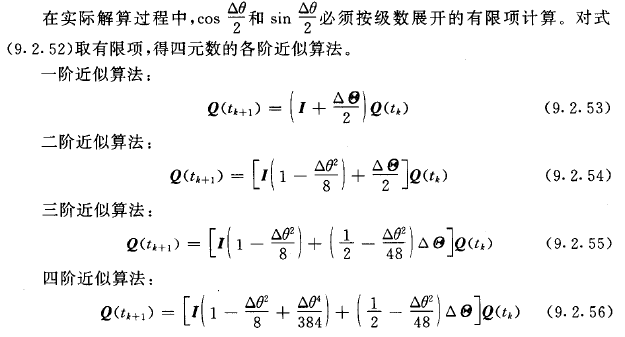其中，
$\Delta \theta^2=\Delta \theta_x^{2}+\Delta \theta_y^{2}+\Delta \theta_z^{2}$

代码如下
void mahony(double currT){
//初始化
static double deltaT_prev=0;
static bool init=false;
if(!init){
deltaT_prev=currT;
init=true;
return ;
}
//**数据准备**
Quaterniond q=curr_pose;//euler2quaternion(align_pose);
Matrix3d Cnb=C_nb_312_from_qnb(q);
Cnb.normalize();

//**对陀螺仪输出角速度进行偏差修正**
Vector3d mag=mag_dev.mag;
mag.normalize();
Vector3d magRef=Cnb.transpose()*mag;  //得到n系下的磁力计分量

Vector3d magRef_(0,sqrt(magRef*magRef+magRef*magRef),magRef);

//估计磁场方向
Vector3d magDir_hat=0.5*Cnb*magRef_;

//mag 与 方向进行叉积
Vector3d mag_cross_magDir=mag.cross(magDir_hat);

//计算偏差
Vector3d err_p(0,0,0);
Vector3d err_i(0,0,0);

double kp_yaw=1.2;
double ki_yaw=0.0002;
err_p=err_p+kp_yaw*mag_cross_magDir;
err_i=err_i+ki_yaw*(currT-deltaT_prev)*mag_cross_magDir;

//**利用加速度计校正pitch、roll偏差计算**
Vector3d acc;
acc=imu_dev.acc;
acc.normalize();

Vector3d vv=Cnb*Vector3d(0,0,1);
Vector3d acc_cross_vv=acc.cross(vv);

double kp_rollpitch=1.2;
double ki_rollpitch=0.0002;
err_p=err_p+kp_rollpitch*acc_cross_vv;
err_i=err_i+ki_rollpitch*(currT-deltaT_prev)*acc_cross_vv;

//**对陀螺仪输出角速度进行偏差修正**
Vector3d gyro=imu_dev.gyro;

gyro=gyro+err_p+err_i;

//**角速度积分，得到角度较小变化**
if((currT-deltaT_prev)>0){
gyro=gyro*(currT-deltaT_prev);
}
else
return;

//**解四元数微分方程**
Matrix4d gyro_mat;
gyro_mat<<  0,    -gyro,   -gyro,   -gyro,
gyro,    0,      gyro,    -gyro,
gyro, -gyro,        0,    gyro,
gyro,  gyro,  -gyro,   0;

double gyro_L2=sqrt(gyro*gyro+gyro*gyro+gyro*gyro);

//**毕卡解法**
Vector4d q_curr;
q_curr<<q.w(),q.x(),q.y(),q.z();
Vector4d q_new=((1-gyro_L2*gyro_L2/8)*Matrix4d::Identity()+(0.5-gyro_L2*gyro_L2/48)*gyro_mat)*q_curr;

Quaterniond output;
output.w()=q_new;
output.x()=q_new;
output.y()=q_new;
output.z()=q_new;
output.normalize();
curr_pose=output;
deltaT_prev=currT;
}

6.4 基于DCM余弦矩阵的姿态解算

基于DMC的解法基本思路与Mahony解法相似，这里不赘述，有兴趣的同学可以参考Razor_AHRS的算法
传送门：https://github.com/KristofRobot/razor_imu_9dof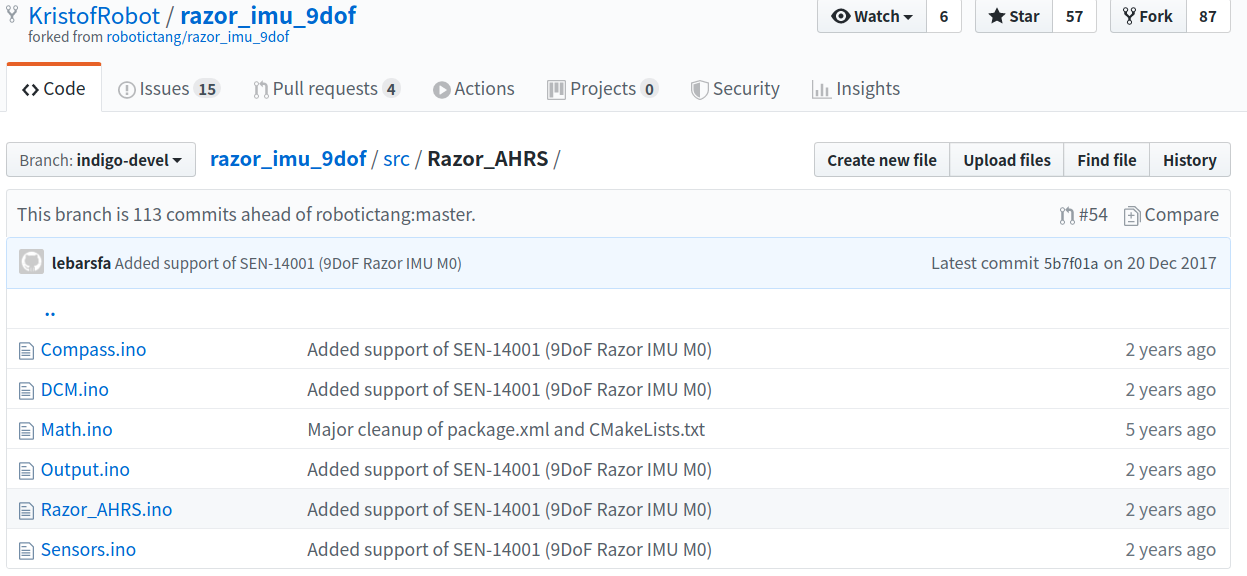6.5 基于扩展卡尔曼滤波的姿态解算

这里不展开卡尔曼、扩展卡尔曼的内容，只是把它当作工具使用

概览
1 状态变量、观测量说明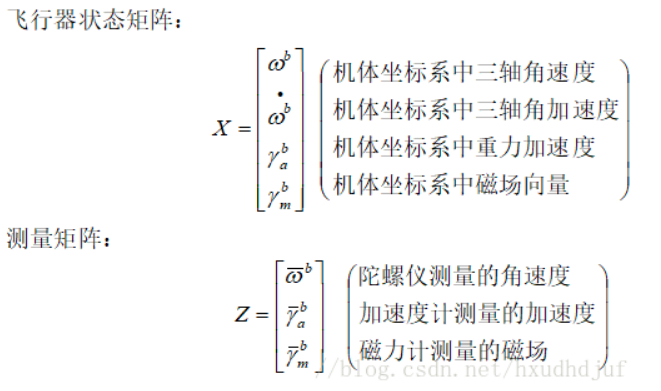2 状态转移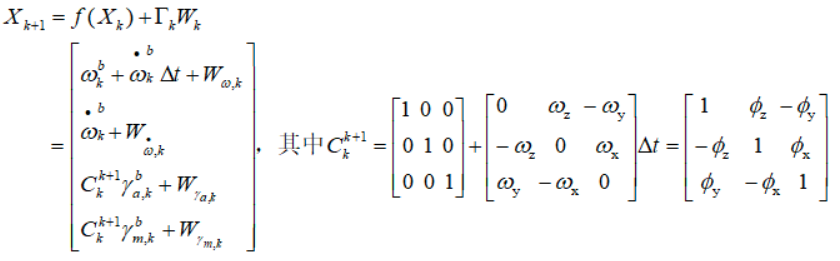3 观测矩阵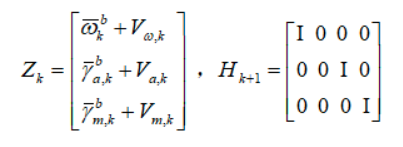4 卡尔曼方程组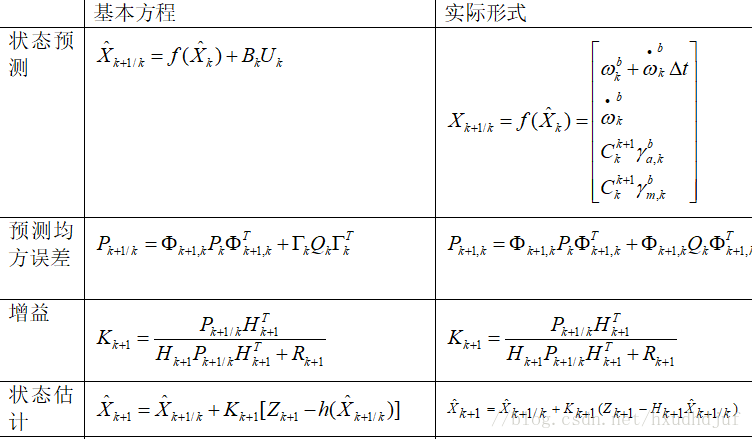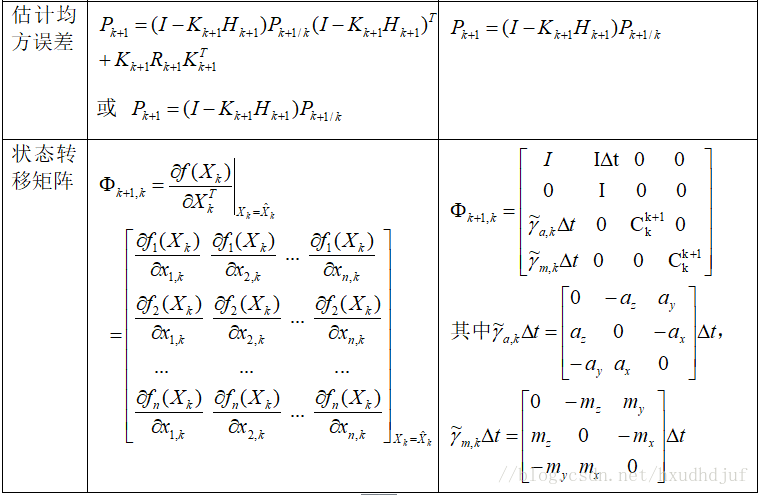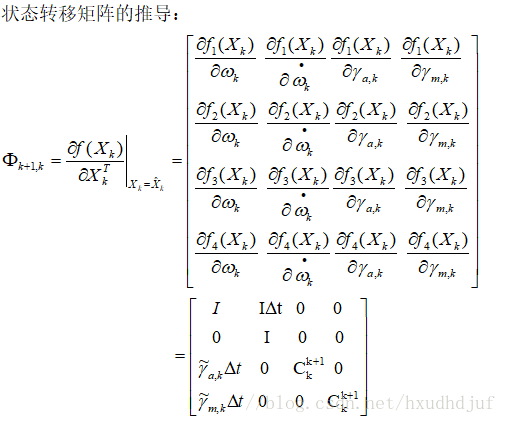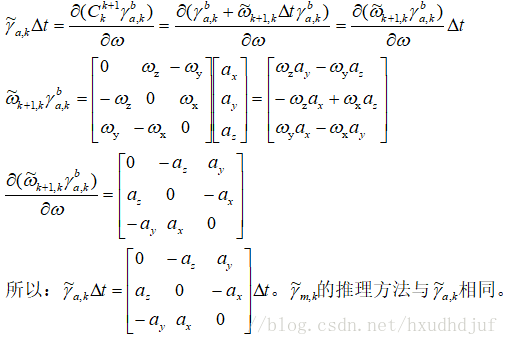概览部分参考自：四旋翼姿态解算–互补滤波和拓展卡尔曼

具体步骤
1 状态变量
\begin{aligned} X_{12x1}= \begin{bmatrix} \hat{gyro}_{3x1} \\ \hat{\dot{gyro}}_{3x1} \\ \hat{Acc}_{3x1}^{b} \\ \hat{Mag}_{3x1}^{b} \end{bmatrix} \begin{bmatrix} b系三轴角速度估计 \\ 角加速度估计 \\ b系加速度估计 \\ b系磁力分量估计 \end{bmatrix} \end{aligned}
2 观测量
\begin{aligned} Z_{9x1}= \begin{bmatrix} gyro_{3x1} \\ Acc_{3x1}^{b} \\ Mag_{3x1}^{b} \end{bmatrix} \begin{bmatrix} b系三轴角速度测量 \\ b系加速度测量 \\ b系磁力分量测量 \end{bmatrix} \end{aligned}
3 状态转移矩阵

非线性状态转移（预测）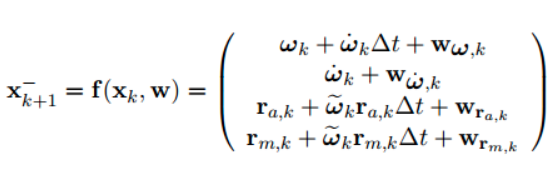其中,
$r_{a,k}为k时刻下的加速度,r_{m,k}为k时刻下的磁力计输出,\tilde w_{k}为描述姿态发生变化的余弦矩阵,W项为高斯噪声$

EKF线性化
对非线性函数在$\hat{x_{k}}$处求一阶导，得到雅克比矩阵为状态转移矩阵
\begin{aligned} A_{lin,k}=\frac{\partial f(x_{k},w_{k})}{\partial x_{k}}= \begin{bmatrix} I_{3x3} &I_{3x3}\Delta T & 0 & 0 \\ 0 &I_{3x3} &0 &0 \\ -r_{acc}\Delta T &0 &I_{3x3}+r_{gyro}\Delta T &0 \\ -r_{mag}\Delta T &0 &0 &I_{3x3}+r_{gyro}\Delta T \end{bmatrix} \end{aligned}
其中,
$r_{acc}$为加速度的反对称矩阵
$r_{mag}$为磁力分量的反对称矩阵
$r_{gyro}$为角速度的反对称矩阵

计算协方差矩阵估计
\begin{aligned} \hat{P}=A_{lin,k}PA_{lin,k}^{T}+Q \end{aligned}

4 更新

观测矩阵
\begin{aligned} H= \begin{bmatrix} I_{3x3} & 0 &0 &0 \\ 0 &0 &I_{3x3} &0\\ 0 &0 &0 &I_{3x3} \end{bmatrix} \end{aligned}
观测
\begin{aligned} Y=Z-H\hat{X} \end{aligned}
计算卡尔曼增益
$K=\hat{P}H^{T}(H\hat{P}H^{T}+R_{k})^{-1}$
更新状态变量估计
$X=\hat{X}+KY$
更新协方差矩阵
$P=(I-KH)\hat{P}$

代码：待更新


展开全文• 标题:vio学习笔记(一) IMU传感器测量原理 在开始介绍IMU这种传感器之前,我们先大体谈一谈VIO的发展背景,VIO是一种视觉惯性估计器,在传统视觉里程计中加入了IMU的信息.那么没什么要加入IMU呢? 原因在于,在单目系统中...
标题:vio学习笔记(一) IMU传感器测量原理
在开始介绍IMU这种传感器之前,我们先大体谈一谈VIO的发展背景,VIO是一种视觉惯性估计器,在传统视觉里程计中加入了IMU的信息.那么没什么要加入IMU呢? 原因在于,在单目系统中,我们很难结算出绝对的尺度信息,这样,我们辛辛苦苦建立的视觉定位系统就无法在实际工程中有良好的应用效果.此外,VO系统还有以下缺点,都使得VIO系统逐步发展起来:
(1)受摄像头本身特点和质量制约,视觉里程计在高速运动,光照条件不良等情况下容易出现算法发散的情况;
(2)视觉里程计系统中我们很难结算出速度信息,而速度参数恰恰是规划路径与控制中不可或缺的指标;
(3)在视觉里程计系统中是没有惯性坐标系的概念的,惯性坐标系通俗的理解就是为了是我们明确重力的方向.如果没有惯性坐标系的概念,视觉里程计系统就有肯能出现定位错误的问题,比如车明明是在水平面上跑,但轨迹却是向天上飞的情况.
此外,视觉传感器与IMU在性能和适用场景上有很多优势互补的地方,视觉传感器适合低速,速度变化慢,纹理丰富的场景,而IMU需要有足够的加速度激励,比较适合高速,速度变化快的场景…视觉传感器受光照影响比较大,而IMU则对光照不敏感.等等,基于以上种种原因,使视觉和IMU的融合成为了各路大神的研究热点,VIO系统也随之诞生.
既然VIO要把IMU信息融入到视觉之中,那么我们首先要知道IMU的工作原理,我们能从IMU中得到什么信息,以及这些信息收到那些噪声的影响等等.
什么是IMU呢?  IMU也成为惯性导航单元,英文名为Inertial Measurement Unit.  其内部由一个加速度计和一个陀螺以组成,分别测量加速度信息和角度信息.各别型号的三轴陀螺仪还会有地磁计.接下来介绍加速度计和陀螺仪的测量原理.
一 MEMS加速度计测量原理
加速度计的测量原理可以用一个简单的弹簧拉力计模型表示.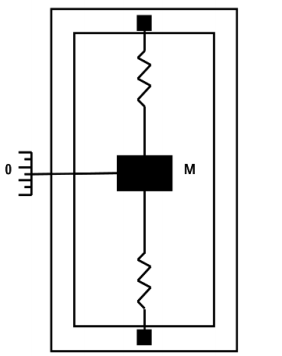加速度的测量值就是拉力计的读数: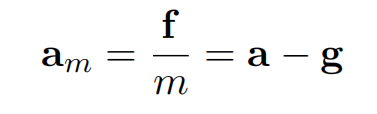a为物体惯性系下的加速度,g为当地的重力加速度.am为拉力计的读数,也就是加速度计的测量值.从公式中也可以看出,物体在惯性系下保持静止状态时,加速度计的测量值在Z轴上应为-g.通常,大多数加速度计同时通过电阻和电阻桥等原理测量的.
二 陀螺仪测量原理
陀螺仪可以分为振动式陀螺仪和光线式陀螺仪,用来测量物体的角速度.光纤陀螺仪成本较高,我们简单介绍一下震动陀螺仪的工作原理.上大学的时候大物课上老师讲过,旋转的物体收到科氏力的作用,震动陀螺仪就是依靠这个原理来测量角度信息的.大概意思就是这个小小的陀螺仪(民用低成本的那种)里有一个质量块,然后这个质量块在正负交替电压的作用下做高速往返运动,当物体有一个旋转时,质量块在往返运动的基础上也会做一个旋转,这就OK了,有了旋转,就会产生科氏力,把科氏力按照大小正比转换成其他信号,比如电流电压啥的,就可以根据科氏力算出角度来,至于算的原理和上面的加速度计类似.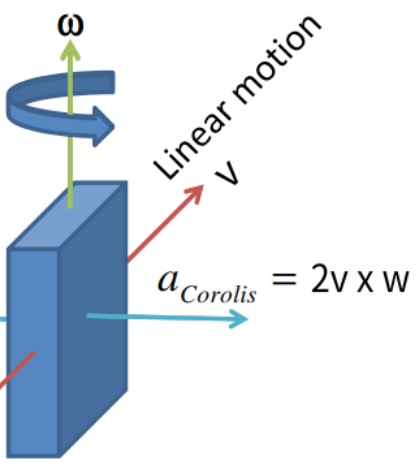IMU测量原理大概就是这个样子,想要详细了解请自行看一些MEMS传感器书籍.不过个人感觉了解这么多就够了.后续会根据测量原理来对IMU进行建模.


展开全文• 作者丨Bjergsen@知乎来源丨https://zhuanlan.zhihu.com/p/68863677编辑丨3D视觉工坊在vio系统中，camera-imu间内外参精确与否对整个定位...算法 计算机视觉 人工智能 机器学习 大数据
• UWB+IMU超宽带和惯导融合定位项目的模块原理图，包括了电源模块（3.0、3.3、5.0）+mcu模块（nrf52832）+加速度计模块（LIS3DH）+DWM1000模块原理
• ## ROS入门：IMU&GPS融合定位实例

千次阅读 热门讨论 2020-07-04 14:53:40
GPS融合定位仿真环境的搭建过程进行讲解，而没有对具体原理的介绍 2。本人作为技术小白，完全参考了https://zhuanlan.zhihu.com/p/152662055的内容（其对环境的搭建讲的较少），对原理感兴趣的童鞋可以参考;若搭建...ros
• 多传感器融合定位（3-惯性导航原理及误差分析）2-IMU误差分析及处理：随机误差理论分析&allan方差分析及实现
• 上一次的分享里，我介绍了GPS的原理（三角定位）及特性（精度、频率），同时也从无人车控制的角度，讨论了为什么仅有GPS无法满足无人车的定位要求。 为了能让无人驾驶系统更高频率地获取定位信息，就必须引入频率更...无人驾驶
• 2.IMU 定位原理 2.1 加速度计 2.2 磁力计 2.3 陀螺仪 3.GPS 定位原理 3.1 直接GPS定位 3.2 差分GPS定位 4.轮速差速航迹推算 4.1 差速器 4.2 航迹推算 5.四元数与姿态解算 5.1 欧拉角 5.2 四元数 6.多重...航迹推算
• 多传感器融合定位（3-惯性导航原理及误差分析）4-IMU温补：常见温补模型与基于多项式你和的温补方法：惯性器件误差分析
• 对于自动驾驶的汽车来说，有时也需要对自身进行更精确的定位，今天就介绍如何使用IMU和GPS进行自车定位（因为在上一篇文章中对kalman和Extend Kalman原理进行了比较详细的介绍，本文中理论部分不会再介绍的这么详细...
• IMUArduIMU + V3是一种非常强大的定位姿态传感器开发板。IMUArduIMU+ V3姿态传感器开发板实物截图: IMU ArduIMU+ V3姿态传感器开发板规格: 3-Axis gyro with sensitivity up to 131 LSBs/dps and a full-scale range...
• ## 无人车传感器 IMU

千次阅读 2019-01-29 09:07:42
IMU（Inertial Measurement Unit）惯性测量单元。为了能让无人驾驶系统更高...IMU原理 当我们晚上回到家，发现家里停电时，眼睛在黑暗中什么都看不见的情况下，只能根据自己的经验，极为谨慎地走小碎步，并不断...
• 为了能让无人驾驶系统更高频率地获取定位信息，就必须引入频率更高的...IMU原理 当我们晚上回到家，发现家里停电时，眼睛在黑暗中什么都看不见的情况下，只能根据自己的经验，极为谨慎地走小碎步，并不断用手摸周
• 相对定位方法的基本原理是在移动机器人位姿初始值给定的前提下，基于内部传感器信息计算出每一时刻位姿相对于上一时刻位姿的距离以及方向角的变化，从而实现位姿的实时估计，该方法也常被人们称作航迹推算方法。...
• 这里简单介绍一下关于惯导的一些进本原理和所用的地方以及imu与ins的区别。 惯性导航通过测量飞行器的加速度，并自动进行积分运算获得飞行器瞬时速度和瞬时位置数据的技术。组成惯性导航系统的设备都安装在运载体内...人工智能
• 在vio系统中，camera-imu间内外参精确与否对整个定位精度起着重要的作用。所以良好的标定结果是定位系统的前提工作。 目前标定算法主要分为离线和在线标定，离线标定以kalibr为代表，能够标定camera内参、camera-imu...
• ## 惯导IMU和惯导INS

千次阅读 2018-08-23 09:18:25
这里简单介绍一下关于惯导的一些进本原理和所用的地方以及imu与ins的区别。   惯性导航 通过测量飞行器的加速度，并自动进行积分运算获得飞行器瞬时速度和瞬时位置数据的技术。组成惯性导航系统的设备都安装在...INS
• 磁力计简介2.1磁力计定位原理2.2 磁力计误差来源（1）自身内部误差（2）应用安装误差（3）罗差3. 磁力计简介 1. 3轴、6轴、9轴IMU的区别 1.1 3轴IMU即只有3轴陀螺仪的IMU，其因为只有一个3轴陀螺仪，所以只能感知...自动驾驶
• 多传感器融合定位（3-惯性导航原理及误差分析）6-Allan方差 进行随机误差分析 参考博客： 组合导航系列文章（四）：Allan方差分析 使用imu_utils工具生成IMU的Allan方差标定曲线 手写VIO-使用Allen方差工具标定IMU ...
• -它介绍了IMU的工作原理，校准背后的数学原理以及寻找侧倾，俯仰和偏航的基本原理。 -该博客深入探讨了卡尔曼滤波器，马达格维克滤波器以及它们在此处的应用背后的数学原理。 -入门概述。 免疫传感器 该仓库提供了...
• IMU的误差模型和标定工作原理加速度计工作原理陀螺仪测量原理误差来源IMU噪声模型biasscale轴偏差IMU校准确定性误差六面法标定bias和scale factor温度相关的参数标定随机误差高斯白噪声Bias 随机游走艾伦方差标定...
• 这里简单介绍一下关于惯导的一些进本原理和所用的地方以及imu与ins的区别。   惯性导航 通过测量飞行器的加速度，并自动进行积分运算获得飞行器瞬时速度和瞬时位置数据的技术。组成惯性导航系统的设备都安装在...INS 惯导
• 上一次的分享里，我介绍了GPS的原理（三角定位）及特性（精度、频率），同时也从无人车控制的角度，讨论了为什么仅有GPS无法满足无人车的定位要求。 为了能让无人驾驶系统更高频率地获取定位信息，就必须引入频率...自动驾驶
• 上一次的分享里，我介绍了GPS的原理（三角定位）及特性（精度、频率），同时也从无人车控制的角度，讨论了为什么仅有GPS无法满足无人车的定位要求。 为了能让无人驾驶系统更高频率地获取定位信息，就必须引入频率更...